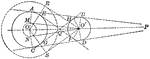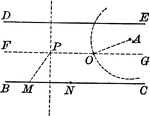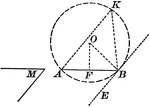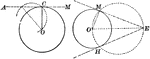### Construction of a Common Tangent When Given Two Circles

Illustration used to construct a common tangent when given two circles.### Construction of a Circle Through a Given Point that Cuts Chords of Given Lengths From Parallels

Illustration used to construct a circle that shall pass through a given point and cut chords of a given…### Construction to Describe a Segment of a Circle in Which an Angle Can Be Inscribed

Illustration of the construction used upon a given straight line, to describe a segment of a circle…### Construction of Tangent Line Through a Given Point to a Given Circle

Illustration of the construction used to draw a tangent to a given circle through a given point.